# ML Aggarwal Solutions Class 10 Maths Chapter 6 Factorization

1. Find the remainder (without division) on dividing f(x) by (x – 2) where
(i) f(x) = 5x2  – 7x + 4
Solutions:-
We have given that:
Let us assume x – 2 = 0
Then, x = 2
Given, f(x) = 5x2  – 7x + 4
Now, substitute the value of x in f(x), we get
f(2)= (5 × 22) – (7 × 2) + 4
= (5 × 4) – 14 + 4
= 20 – 14 + 4
= 24 – 14
= 10
Therefore, the remainder is 10.

(ii) f(x) = 2x3  – 7x2+ 3
Solution:-

Let us assume x – 2 = 0
Then, x = 2
We have given that: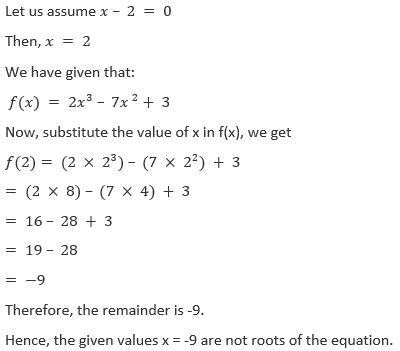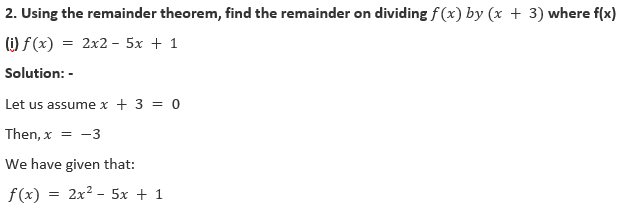Therefore, the remainder is -2
Hence, the given values x = -2 are not roots of the equation.

3. Find the remainder (without division) on dividing f(x) by (2x + 1) where,
(i) f(x) = 4x2  + 5x + 3
Solution:-
Let us assume 2x + 1 = 0
Then, 2x = -1
x=-1/2
We have given that:4. (i) find the remainder (without division) when 2x3  – 3x2  + 7x – 8 is divided by x – 1.
Solution:-
Let us assume x – 1 = 0
Then, x = 1
We have given that:
f(x) = 2x3  – 3x2  + 7x – 8
Now, substitute the value of x in f(x), we get
f(1) = (2 × 13) – (3 × 12) + (7 × 1) – 8
= 2 – 3 + 7 – 8
= 9 – 11
= – 2
Hence, the given values x = -2 are not roots of the equation.

(ii) Find the remainder (without division) on dividing 3x2  + 5x – 9 by (3x + 2).
Solution:-
Let us assume 3x + 2 = 0
3x = -2
x = -2/3
Then, x = -2/3
We have given that:
f(x) = 3x2  + 5x – 9
Now, substitute the value of x in f(x), we get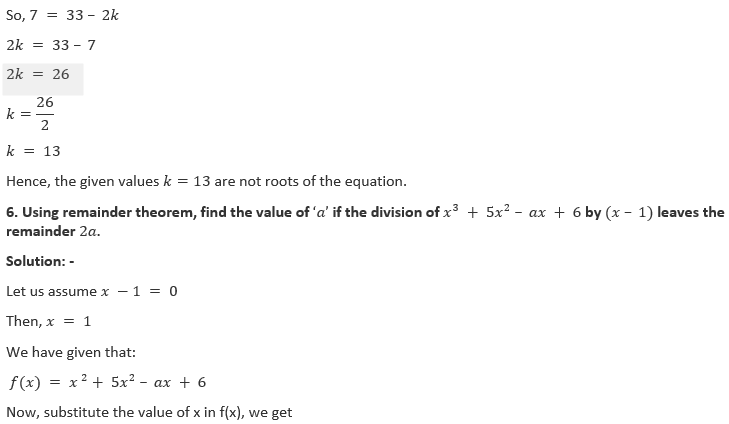f(1) = 13+ (5 × 12) – (a × 1) + 6
= 1 + 5 – a + 6
= 12 – a
From the question it is given that, remainder is 2a
So, 2a = 12 – a
2a + a = 12
3a = 12
a = 12/3
a = 4
Hence, the given values a=4 are not roots of the equation.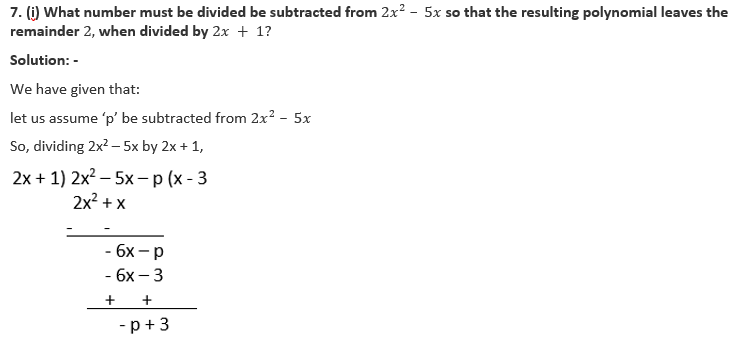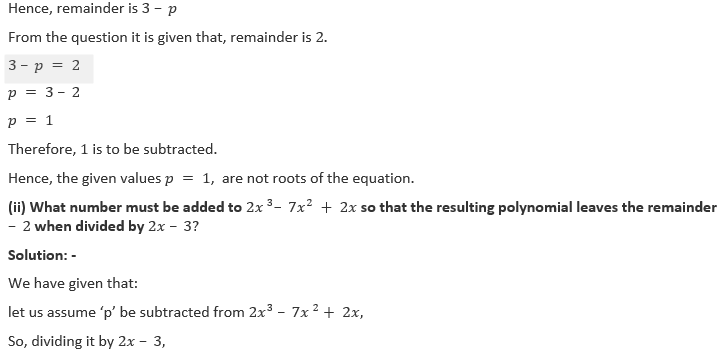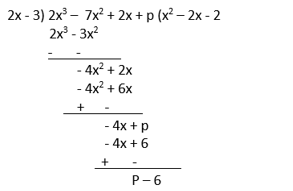Hence, remainder is p – 6
From the question it is given that, remainder is – 2.
P – 6 = – 2
P = -2 + 6
P = 4
Hence, the given values p=4 are not roots of the equation.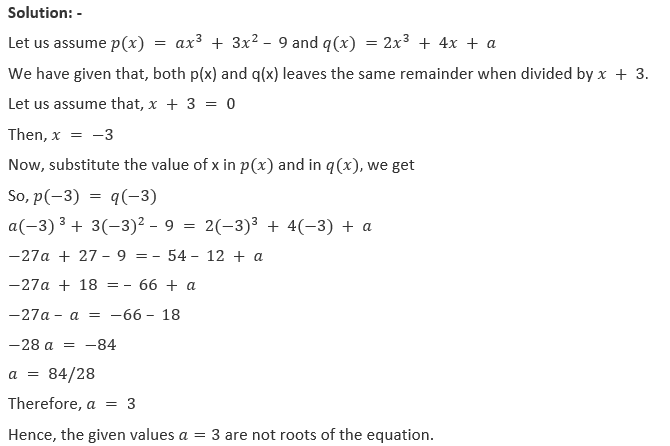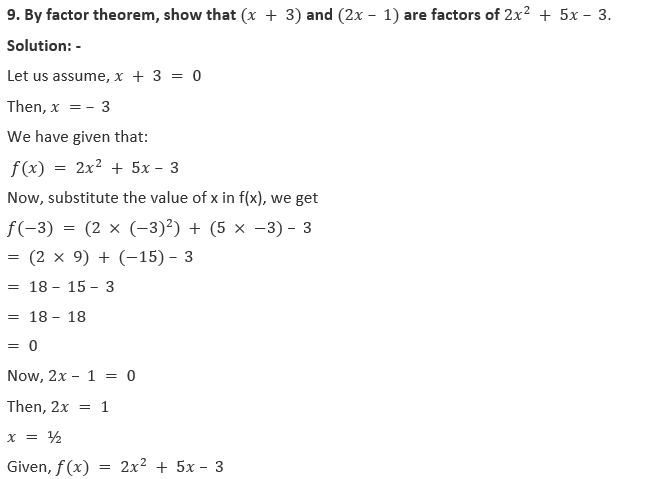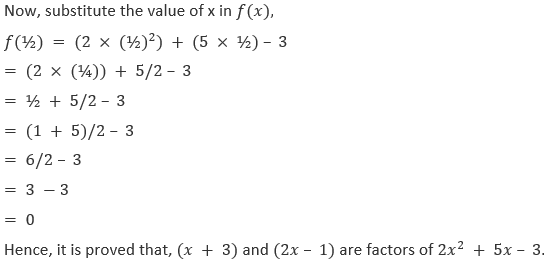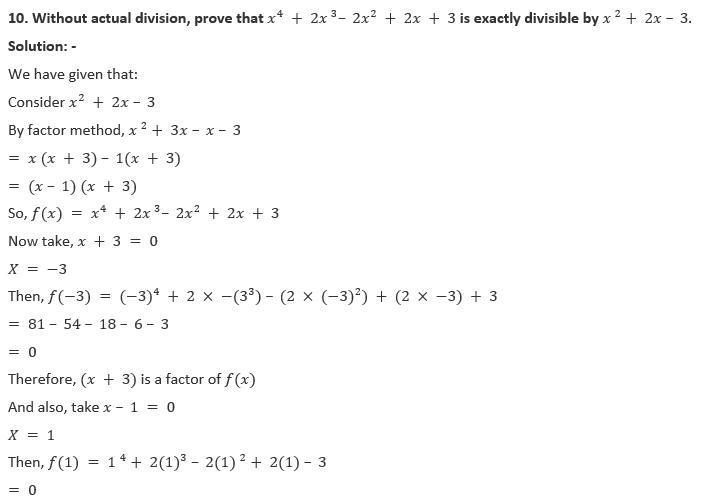x = -7/2
We have given that:
f(x) = 2x3  + 5x 2– 11x – 14
Now, substitute the value of x in f(x), we get
f(-7/2) = 2(-7/2)3+ 5(-7/2)2+ 11(-7/2) – 14
= 2(-343/8) + 5(49/4) + (-77/2) – 14
= -343/4 + 245/4 – 77/2 – 14
= (-343 + 245 + 154 – 56)/4
= -399 + 399/4
= 0
Therefore, (2x + 7) is a factor of 2x3  + 5x2  – 11x – 14
Then, dividing f(x) by (2x + 1), we get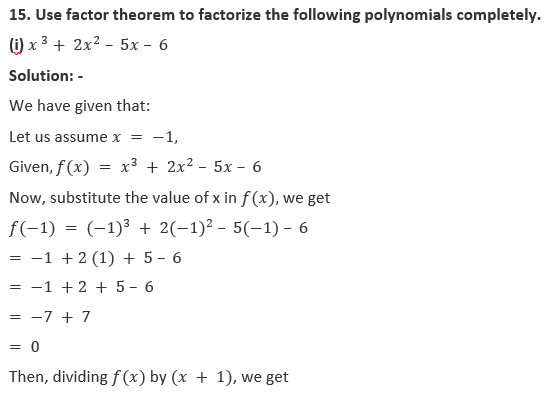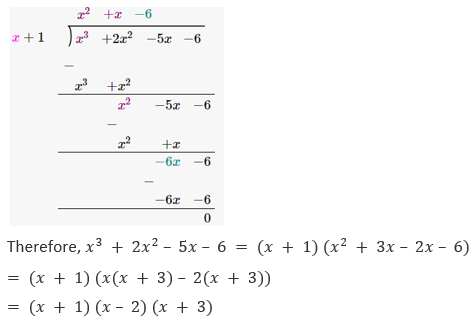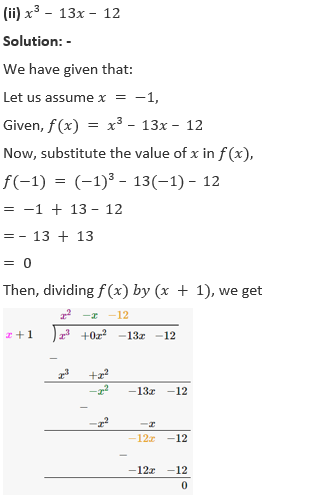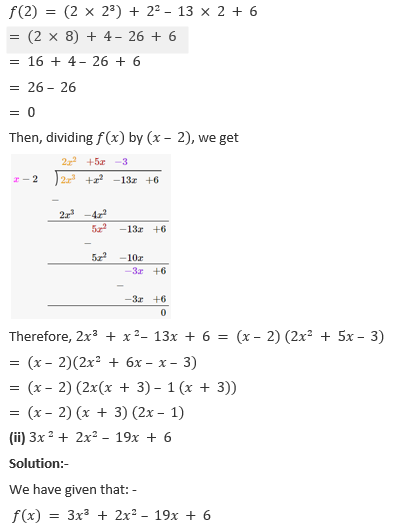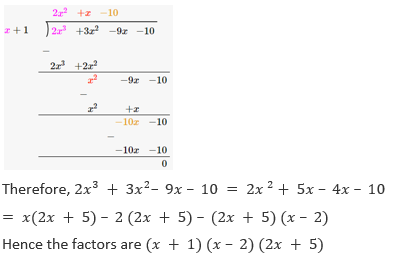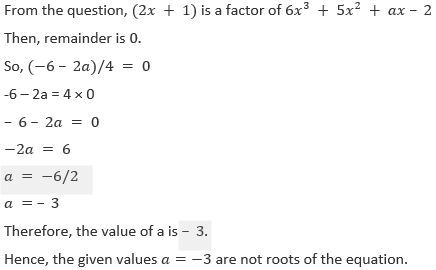Then, remainder is 0
So, (44 – 4k)/9 = 0
44 – 4k = 0 × 9
44 = 4k
K = 44/4
K = 11
Hence, the value of k is 11.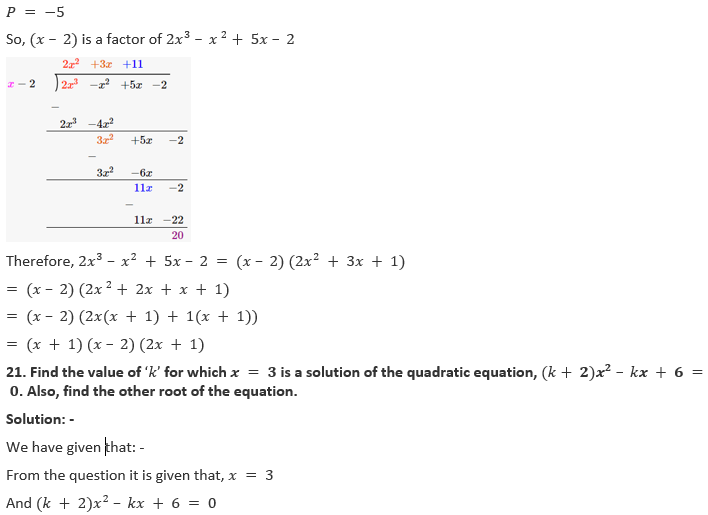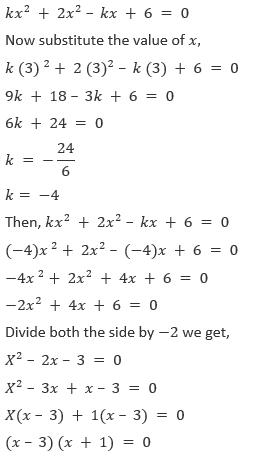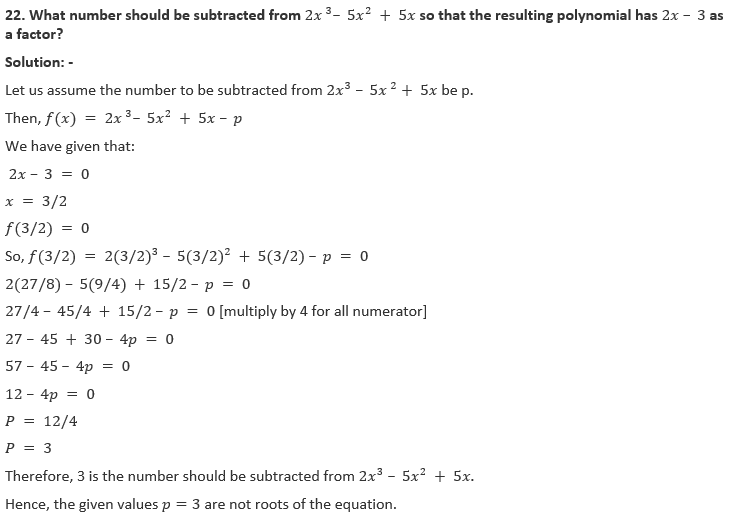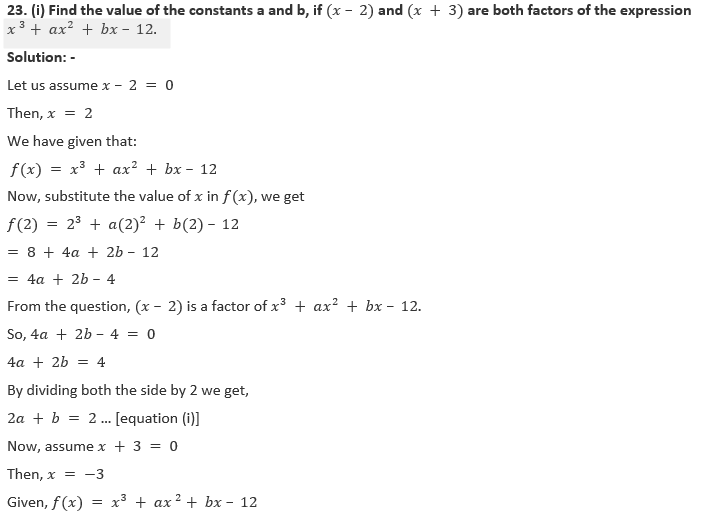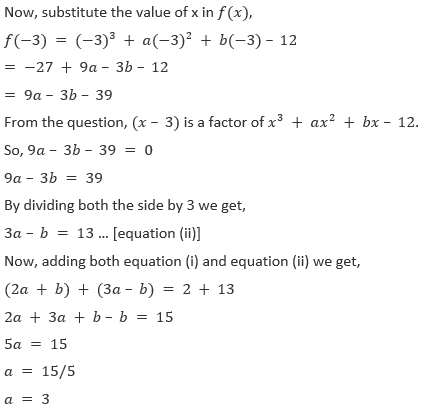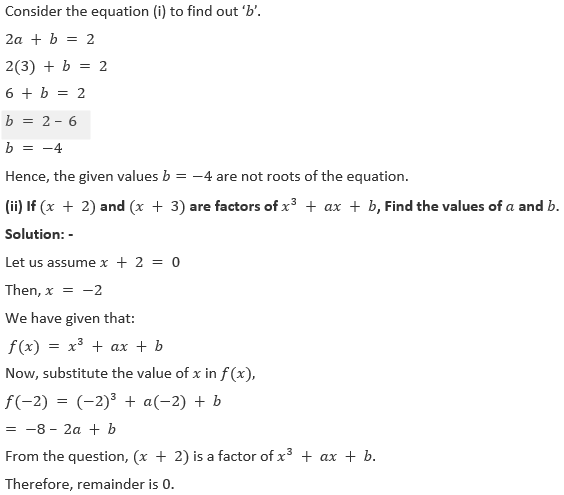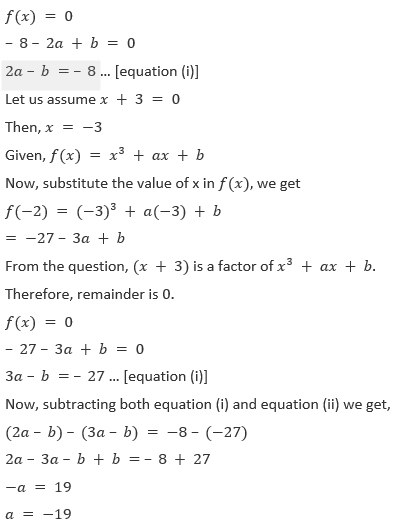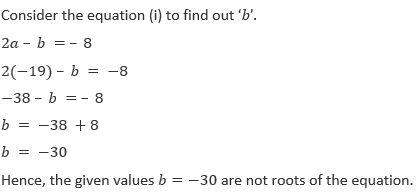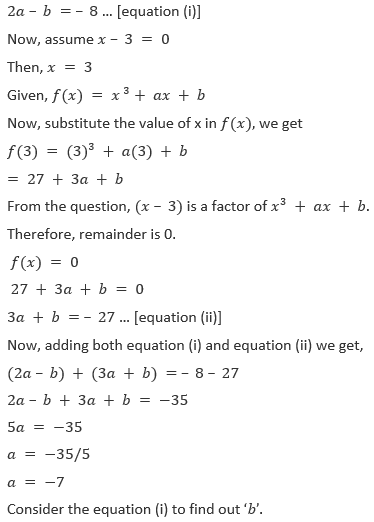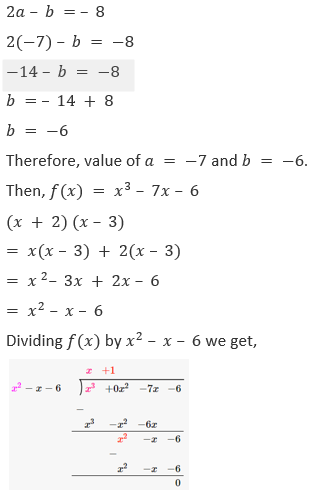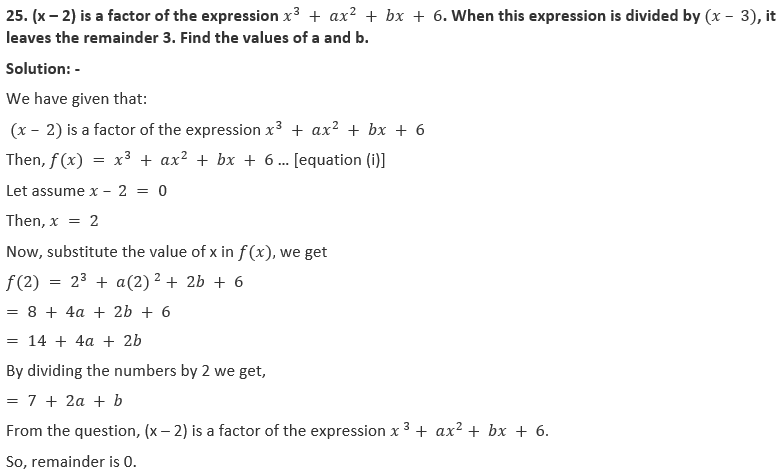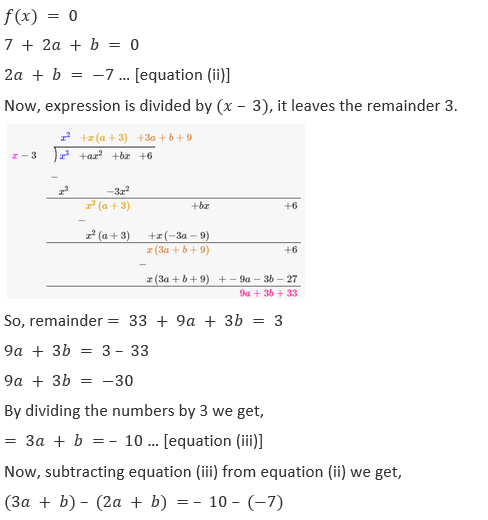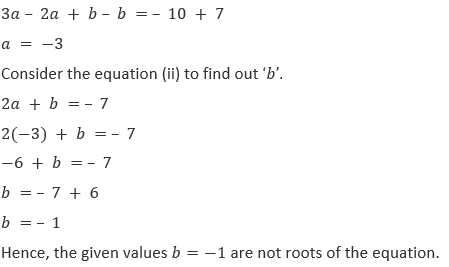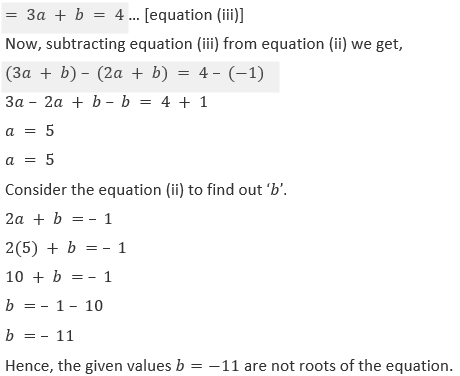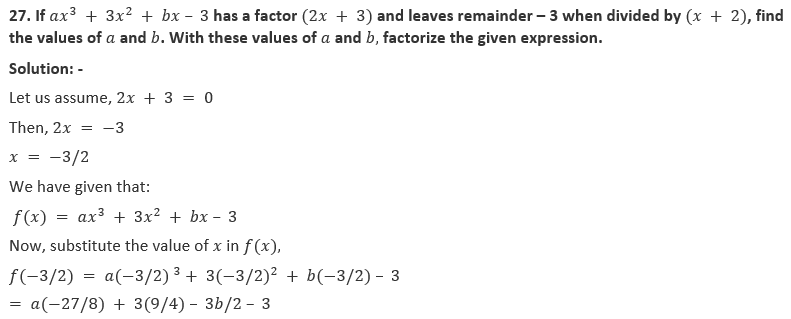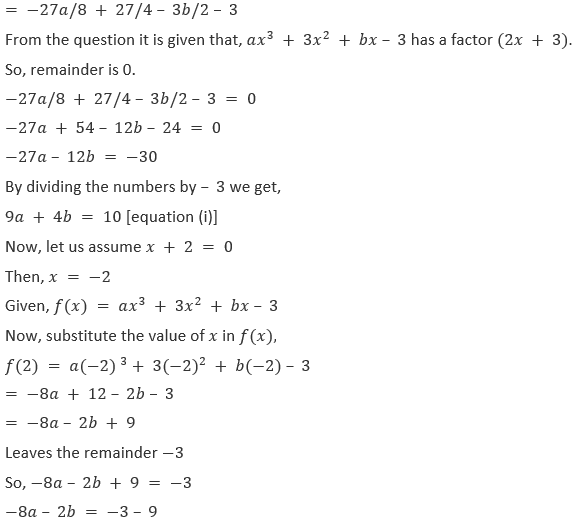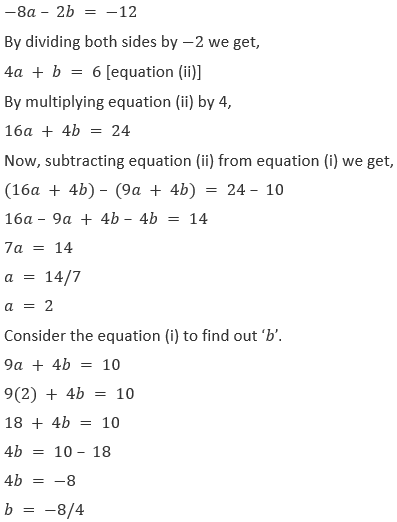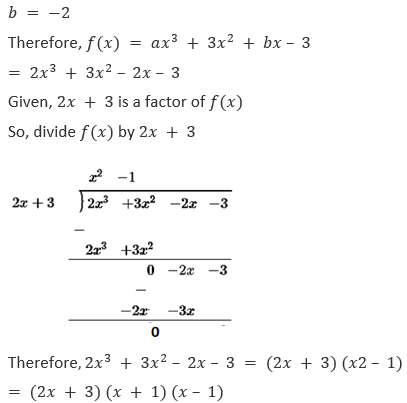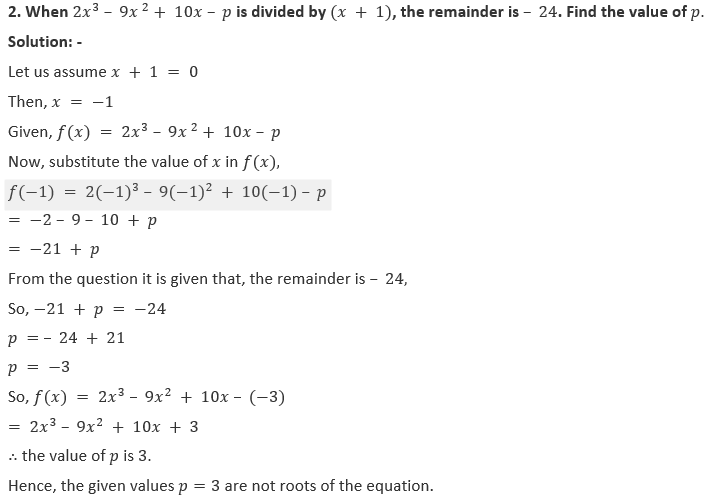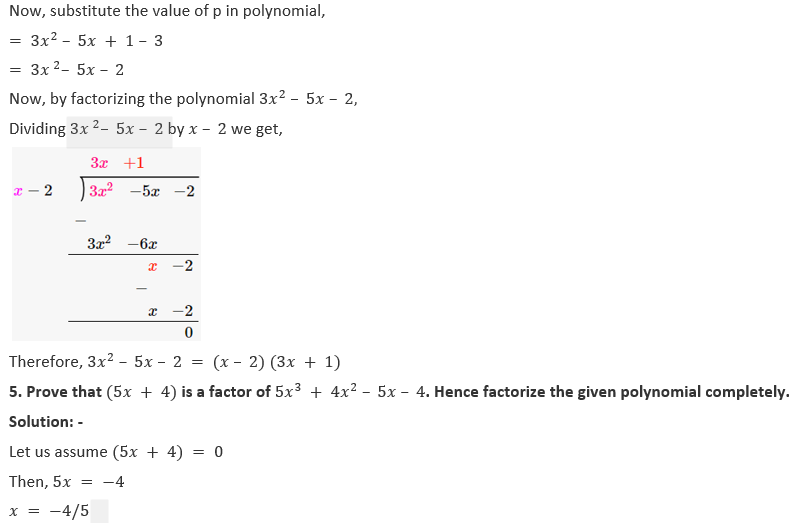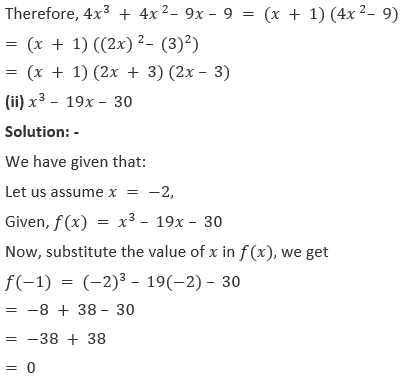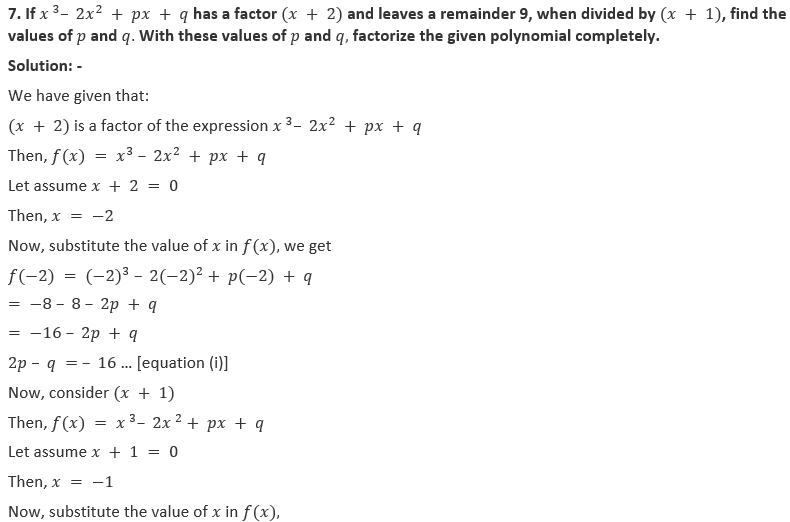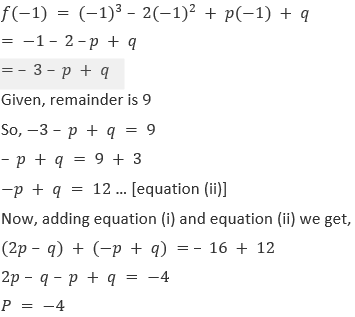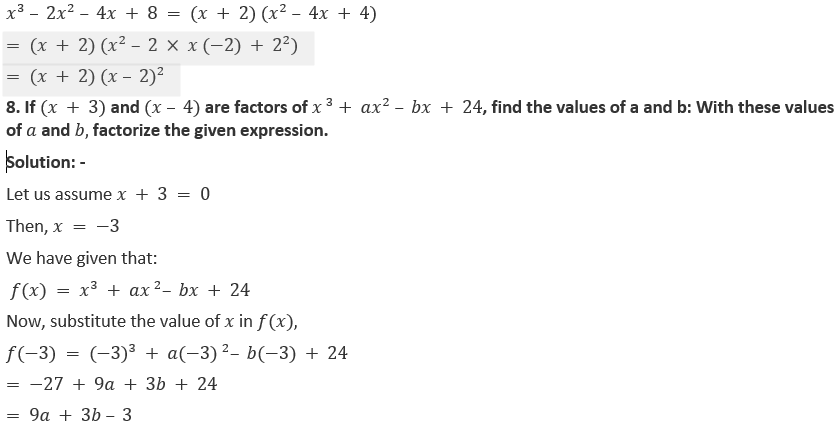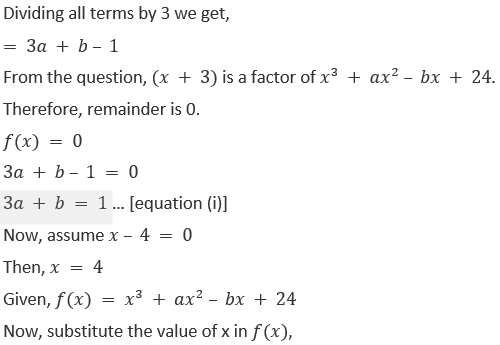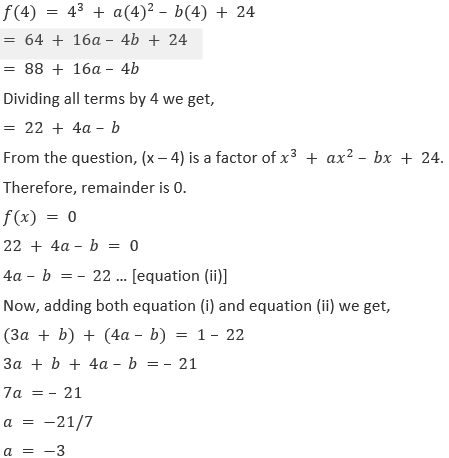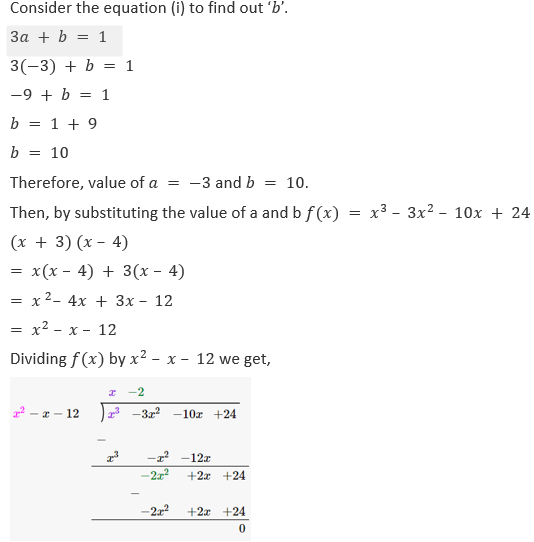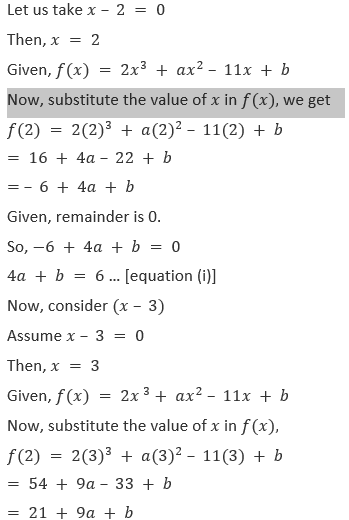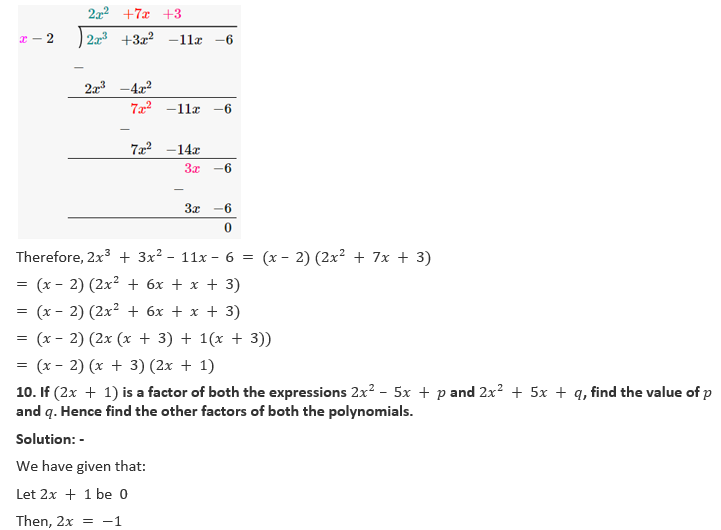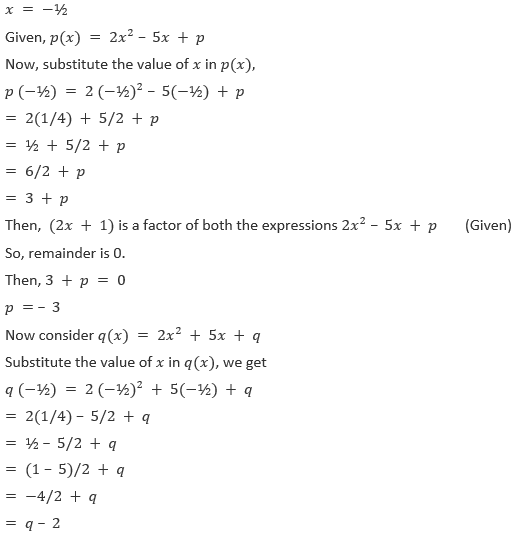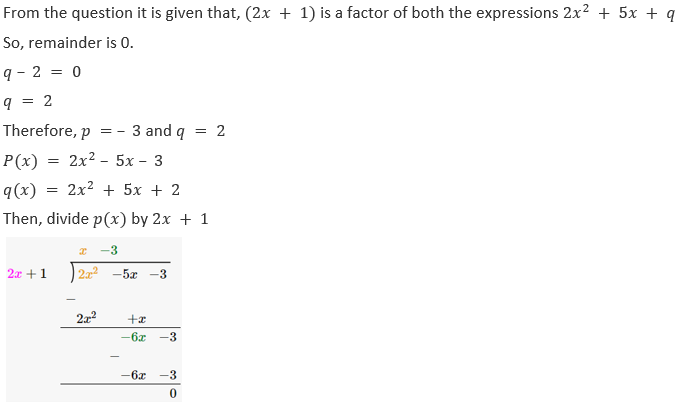2 – a + b = 5
– a + b = 5 – 2
– a + b = 3 … (ii)
Now, subtracting equation (ii) from equation (i) we get,
(a + b) – (- a + b) = 13 – 3
a + b + a – b = 10
2a = 10
a = 10/2
a = 5
To find out the value of b, substitute the value of a in equation (i) we get,
a + b = 13
5 + b = 13
b = 13 – 5
b = 8
Therefore, value of a = 5 and b = 8
Hence, the given values b=8 are not roots of the equation.

12. When a polynomial f(x) is divided by (x – 1), the remainder is 5 and when it is, divided by (x – 2), the remainder is 7. Find the remainder when it is divided by (x – 1) (x – 2).
Solution:-
We have given that:
Polynomial f(x) is divided by (x – 1),
Remainder = 5
Let  x – 1 be  0
x = 1
f(1) = 5
and the divided be (x – 2), remainder = 7
let us assume x – 2 = 0
x = 2
Therefore, f(2) = 7
/So, f(x) = (x – 1) (x – 2) q(x) + ax + b
Where, q(x) is the quotient and ax + b is remainder,
Now put x = 1, we get,
f(1) = (1 – 1)(1 – 2)q(1) + (a × 1) + b
a + b = 5 … (i)
x = 2,
f(2) = (2 – 1)(2 – 2)q(2) + (a × 2) + b
2a + b = 7 … (ii)
Now subtracting equation (i) from equation (ii) we get,
(2a + b) – (a + b) = 7 – 5
2a + b – a – b = 2
a = 2
To find out the value of b, substitute the value of a in equation (i) we get,
a + b = 5
2 + b = 5
b = 5 – 2
b = 3
Hence, the remainder ax+b=2x+3